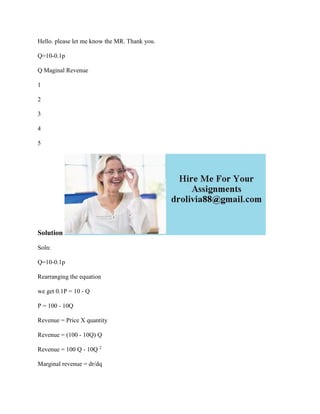Seu SlideShare está sendo baixado. ×

# Hello- please let me know the MR- Thank you- Q-10-0-1p Q Maginal Reven.docx

Anúncio
Anúncio
Anúncio
Anúncio
Anúncio
Anúncio
Anúncio
Anúncio
Anúncio
Anúncio
AnúncioCarregando em…3
×

1 de 2 Anúncio

# Hello- please let me know the MR- Thank you- Q-10-0-1p Q Maginal Reven.docx

Hello. please let me know the MR. Thank you.
Q=10-0.1p
Q Maginal Revenue
1
2
3
4
5
Solution
Soln:
Q=10-0.1p
Rearranging the equation
we get 0.1P = 10 - Q
P = 100 - 10Q
Revenue = Price X quantity
Revenue = (100 - 10Q) Q
Revenue = 100 Q - 10Q 2
Marginal revenue = dr/dq
dr/dq = 100 - 20Q

.

Hello. please let me know the MR. Thank you.
Q=10-0.1p
Q Maginal Revenue
1
2
3
4
5
Solution
Soln:
Q=10-0.1p
Rearranging the equation
we get 0.1P = 10 - Q
P = 100 - 10Q
Revenue = Price X quantity
Revenue = (100 - 10Q) Q
Revenue = 100 Q - 10Q 2
Marginal revenue = dr/dq
dr/dq = 100 - 20Q

.

Anúncio
Anúncio

### Hello- please let me know the MR- Thank you- Q-10-0-1p Q Maginal Reven.docx

1. 1. Hello. please let me know the MR. Thank you. Q=10-0.1p Q Maginal Revenue 1 2 3 4 5 Solution Soln: Q=10-0.1p Rearranging the equation we get 0.1P = 10 - Q P = 100 - 10Q Revenue = Price X quantity Revenue = (100 - 10Q) Q Revenue = 100 Q - 10Q 2 Marginal revenue = dr/dq
2. 2. dr/dq = 100 - 20Q Q Marginal revenue 1 80 2 60 3 40 4 20 5 0